# Brass

Brass is an alloy of copper and zinc in a ratio of 3: 2. How many grams does a component that required 270 g of copper weigh?

m =  450 g

### Step-by-step explanation: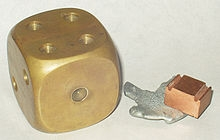Did you find an error or inaccuracy? Feel free to write us. Thank you!Tips to related online calculators
Need help to calculate sum, simplify or multiply fractions? Try our fraction calculator.
Check out our ratio calculator.

## Related math problems and questions:

• RatiosReduce the numbers: 50 in a 1:2 ratio 111 at a ratio of 2:3 70 at 10:50 560 at a ratio of 3:8
• Land areaThe land area of Asia and Africa are in a 3: 2 ratio, the European and African are is 1:3. What are the proportions of Asia, Africa, and Europe?
• CuZn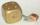Brass is an alloy of copper and zinc. The 10 centimeter brass cube has a weight of 8.6 kg. Copper density is 8930 kg/m3, the zinc density is 7130 kg/m3. Calculate how many kg of copper and zinc a cube contains.
• Electric energyThere are 875 identical light bulbs in the sports hall lighting for 2 hours. How long does the same amount of electricity consume 100 such bulbs?
• Buns and toastsTwo buns weigh 10 grams more than two toasts. One Bun and two Toast weigh a total of 110 grams. How many grams weigh three toasts? How many grams does a Bun weigh?
• Alloy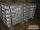The first alloy is a mixture of two metals in the ratio 1:2, the second is a mixture of same metals in the ratio 2:3. At what ratio we have these two alloys put into the furnace to obtain a new metal alloy with ratio 17:27? (All three ratios correspond to
• Two discounts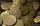The MP4 player cost 960 CZK, the price was twice gradually reduced in the ratio 4: 3: 3/2. How much did the player cost after the second discount?
• Two-fifths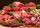Two-fifths (2/5) of a pound of chicken for each person. If there are 8 people to be fed, how many pounds of chicken are required?
• Kilo candyOne kilogram of chocolate candy cost 10 euros. Kate buys 250 grams and had the 8 pack of candy. How much does cost one bonbon?
• DivideDivide the number 72 in the ratio 7: 2 and calculate the ratio of the numbers found in this order and write down as decimal.
• In theIn the national park, the ratio of the wooded area to grassland is 4: 1. The total area is 385km2. What area is wooded?
• ErikThree friends Peter, Erik and Milan shared 420 euros in a ratio of 1: 2: 3. How many euros did Erik get?
• Euros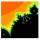Michal, Peter, John and Lenka got together 2,400 euros. They share an amount in ratio 2:6:4:3. How many got each of them?
• BuildingAt the building, we divided 240 boards into two piles in a 5: 3 ratio. How many were fewer boards in the lower pile?
• Composite ratioJakub, Aneta, and Lenka divided 1342 USD in the ratio 5/2: 3/10: 1/4. How much did Lenka take?
• Three numbers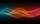The sum of the three numbers is 287. These numbers are in a ratio of 3: 7: 1/4. Find these numbers.
• Carbon dioxide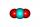Calculate how many grams of oxygen are in 50 g of carbon dioxide CO2. Relative atomic mass of oxygen is 16 and of carbon is 12.